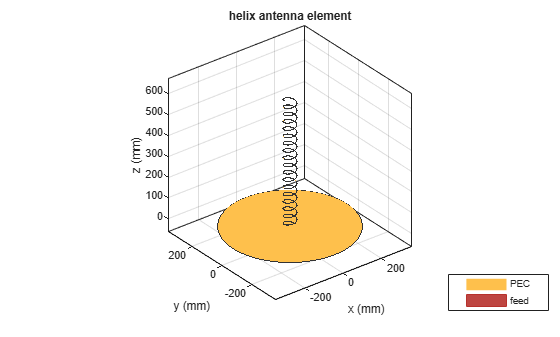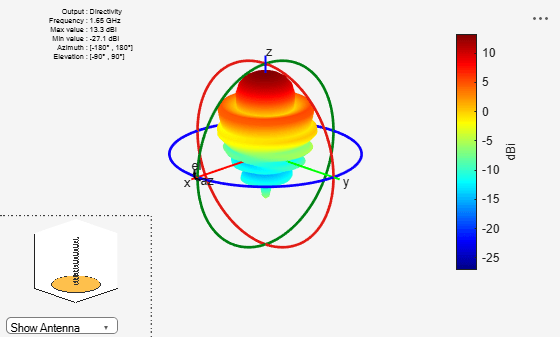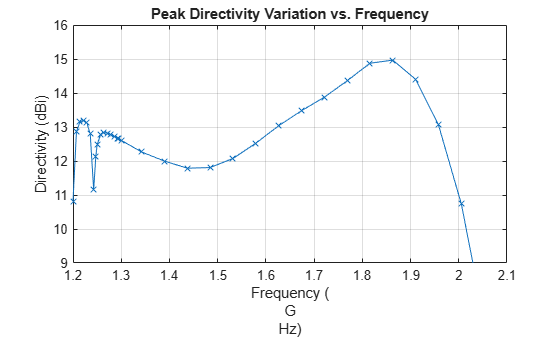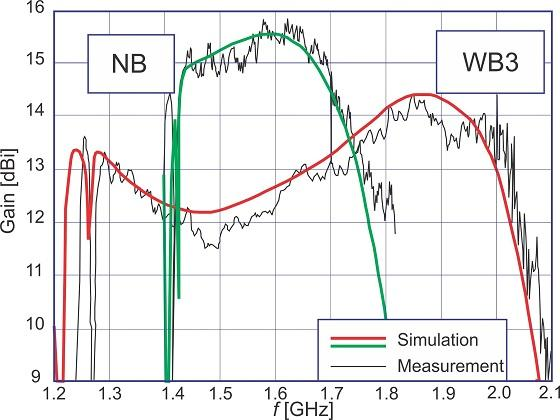# Helical Antenna Design

This example studies a helical antenna designed in  with regard to the achieved directivity. Helical antennas were introduced in 1947 . Since then, they have been widely used in certain applications such as mobile and satellite communications. Helical antennas are commonly used in an axial mode of operation which occurs when the circumference of the helix is comparable to the wavelength of operation. In this mode, the helical antenna has the maximum directivity along its axis and radiates a circularly-polarized wave.

### Helix Design Specifications

The helical antenna design specifications are as follows ():

• Frequency range: 1.3 - 2 GHz

• Gain: 13 dBi +/- 1.5 dBi

• Axial Ratio: < 1.5

### Model Assumptions and Differences

Compared to Ref. , the helical antenna model available in the toolbox uses the following simplifying assumptions:

• Conductor - The original reference uses a cylinder of radius r while the toolbox uses a strip of width w.

• Ground plane shape - A square ground plane is used in the original reference, while the present toolbox model uses a circular shape.

• Width of Feed - The width of the feed in  is r/10 while the toolbox model uses w.

• Design validation metric - Ref.  used the gain to compare simulation and measurement results, whereas in the toolbox, we will use the directivity since the simulated antenna has a negligibly small loss.

### Helix Design Parameters

The helix model in the toolbox uses the strip approximation which relates the width of the strip to the radius of an equivalent cylinder . In addition, the helix model in the toolbox has a circular ground plane. Choose the radius of the ground plane to be half the length of side of the square ground plane.

```r = 0.3e-3; width = cylinder2strip(r); feedheight = 3*r; D = 56e-3; radius = D/2; turns = 17.5; pitch = 11.2; spacing = helixpitch2spacing(pitch,radius); side = 600e-3; radiusGP = side/2;```

### Frequency of Operation and Bandwidth

The center frequency is chosen as 1.65 GHz. A relative bandwidth of 45% is chosen which provides sufficient flexibility since the operating frequency limits result in a relative bandwidth of 42.5%. Relative bandwidth is calculated as,

`$B{W}_{relative}=\left({f}_{upper}-{f}_{lower}\right)/{f}_{c}$`

```fc = 1.65e9; relativeBW = 0.45; BW = relativeBW*fc;```

### Create Helical Antenna

Create the helix antenna with the appropriate properties as calculated before and view the structure.

```hx = helix('Radius',radius,'Width',width,'Turns',turns,... 'Spacing',spacing,'GroundPlaneRadius',radiusGP,... 'FeedStubHeight',feedheight); figure; show(hx);```### Pattern Behavior

Plot the directivity radiation pattern of the helical antenna at the center frequency of 1.65 GHz. This pattern confirms the axial mode of operation for the helical antenna.

```figure; pattern(hx,fc);```To calculate the directivity variation of the main beam as a function of frequency, choose a frequency range according to .

```Nf1 = 15; Nf2 = 20; fmin = 1.2e9; fmax = 2.1e9; fstep = 0.1e9; fband1 = linspace(fmin,1.3e9,Nf1); fband2 = linspace(fmin,fmax,Nf2); freq = unique([fband1,fband2]); Nf = length(freq); D = nan(1,Nf); f_eng = freq./1e9; f_str = 'G'; fig1 = figure; for i = 1:length(freq) D(i) = pattern(hx,freq(i),0,90); figure(fig1) plot(f_eng,D,'x-') grid on axis([f_eng(1) f_eng(end) 9 16 ]) xlabel(['Frequency (' f_str 'Hz)']) ylabel('Directivity (dBi)') title('Peak Directivity Variation vs. Frequency') drawnow end```### Discussion on the Results

Comparing this result with Fig. 11 in  replicated below, we establish the quantitative agreement.Simulated and measured RHC gain for the NB and WB3 designs  (Reproduced with permission from IEEE)